Publicité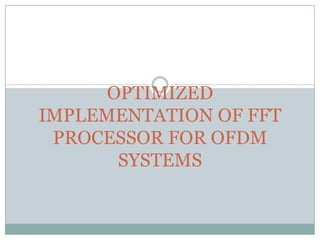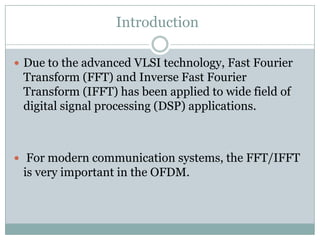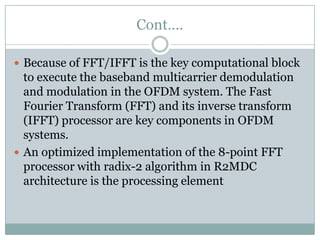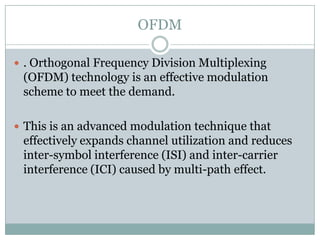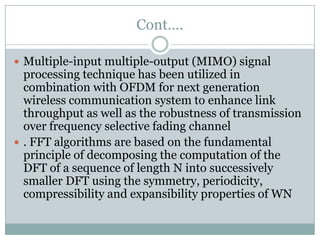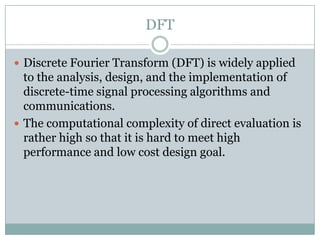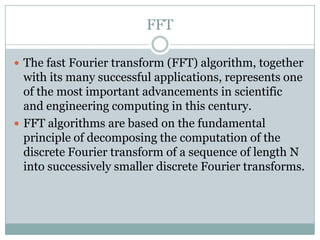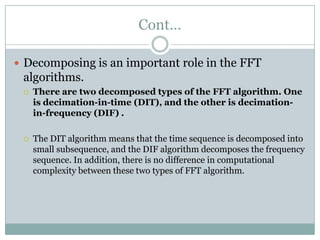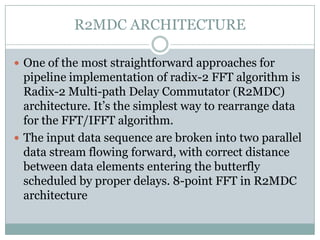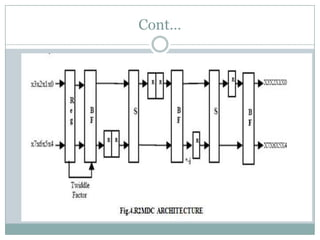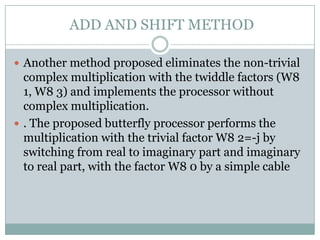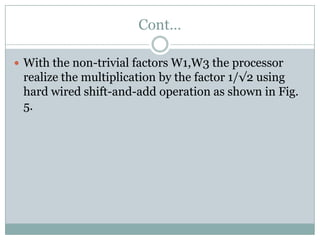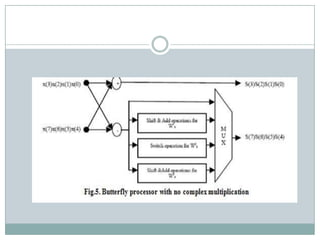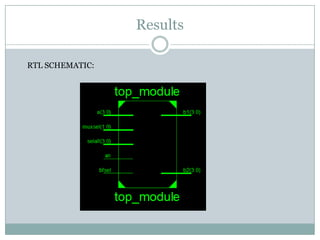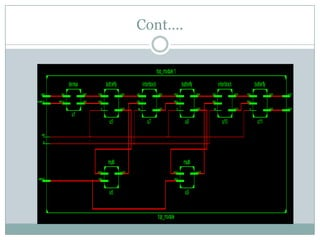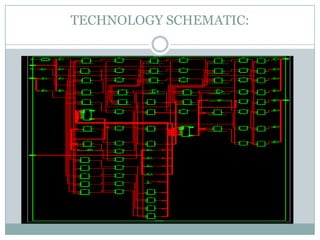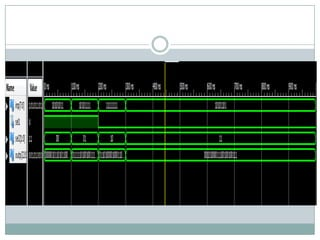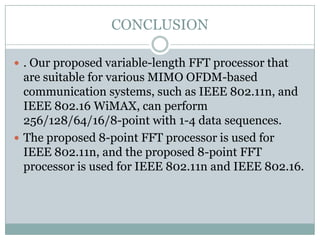1 sur 19
Publicité

### Fft

1. OPTIMIZED IMPLEMENTATION OF FFT PROCESSOR FOR OFDM SYSTEMS
2. Introduction  Due to the advanced VLSI technology, Fast Fourier Transform (FFT) and Inverse Fast Fourier Transform (IFFT) has been applied to wide field of digital signal processing (DSP) applications.  For modern communication systems, the FFT/IFFT is very important in the OFDM.
3. Cont….  Because of FFT/IFFT is the key computational block to execute the baseband multicarrier demodulation and modulation in the OFDM system. The Fast Fourier Transform (FFT) and its inverse transform (IFFT) processor are key components in OFDM systems.  An optimized implementation of the 8-point FFT processor with radix-2 algorithm in R2MDC architecture is the processing element
4. OFDM  . Orthogonal Frequency Division Multiplexing (OFDM) technology is an effective modulation scheme to meet the demand.  This is an advanced modulation technique that effectively expands channel utilization and reduces inter-symbol interference (ISI) and inter-carrier interference (ICI) caused by multi-path effect.
5. Cont….  Multiple-input multiple-output (MIMO) signal processing technique has been utilized in combination with OFDM for next generation wireless communication system to enhance link throughput as well as the robustness of transmission over frequency selective fading channel  . FFT algorithms are based on the fundamental principle of decomposing the computation of the DFT of a sequence of length N into successively smaller DFT using the symmetry, periodicity, compressibility and expansibility properties of WN
6. DFT  Discrete Fourier Transform (DFT) is widely applied to the analysis, design, and the implementation of discrete-time signal processing algorithms and communications.  The computational complexity of direct evaluation is rather high so that it is hard to meet high performance and low cost design goal.
7. FFT  The fast Fourier transform (FFT) algorithm, together with its many successful applications, represents one of the most important advancements in scientific and engineering computing in this century.  FFT algorithms are based on the fundamental principle of decomposing the computation of the discrete Fourier transform of a sequence of length N into successively smaller discrete Fourier transforms.
8. Cont…  Decomposing is an important role in the FFT algorithms.  There are two decomposed types of the FFT algorithm. One is decimation-in-time (DIT), and the other is decimation- in-frequency (DIF) .  The DIT algorithm means that the time sequence is decomposed into small subsequence, and the DIF algorithm decomposes the frequency sequence. In addition, there is no difference in computational complexity between these two types of FFT algorithm.
9. R2MDC ARCHITECTURE  One of the most straightforward approaches for pipeline implementation of radix-2 FFT algorithm is Radix-2 Multi-path Delay Commutator (R2MDC) architecture. It’s the simplest way to rearrange data for the FFT/IFFT algorithm.  The input data sequence are broken into two parallel data stream flowing forward, with correct distance between data elements entering the butterfly scheduled by proper delays. 8-point FFT in R2MDC architecture
10. Cont…
11. ADD AND SHIFT METHOD  Another method proposed eliminates the non-trivial complex multiplication with the twiddle factors (W8 1, W8 3) and implements the processor without complex multiplication.  . The proposed butterfly processor performs the multiplication with the trivial factor W8 2=-j by switching from real to imaginary part and imaginary to real part, with the factor W8 0 by a simple cable
12. Cont…  With the non-trivial factors W1,W3 the processor realize the multiplication by the factor 1/√2 using hard wired shift-and-add operation as shown in Fig. 5.
13. Results RTL SCHEMATIC:
14. Cont….
15. TECHNOLOGY SCHEMATIC:
16. DESIGN SUMMARY:
17. CONCLUSION  . Our proposed variable-length FFT processor that are suitable for various MIMO OFDM-based communication systems, such as IEEE 802.11n, and IEEE 802.16 WiMAX, can perform 256/128/64/16/8-point with 1-4 data sequences.  The proposed 8-point FFT processor is used for IEEE 802.11n, and the proposed 8-point FFT processor is used for IEEE 802.11n and IEEE 802.16.
Publicité